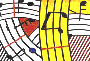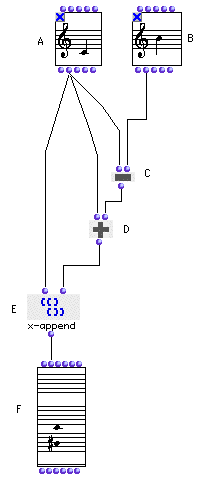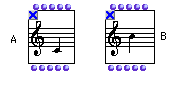Tutorial 2 - Inversion of an interval (I)

Topics

Note inversion using arithmetical operators.

Functions used

NOTE,om+, om-, and x-append.Description

This patch returns the inversion of an interval in the NOTE box (A). That is to say that if you put the interval C3-G3 (an interval of a fifth) it will return the interval F2-C3.

Patch structure

A: Open the NOTE box (A) by clicking in the center of the box. Edit the lower note of your interval. If, for instance, you want a major seventh C-B, the lower note is C. Then close it and, don't forget to lock it.B: Do the same thing in the NOTE box (B), this time editing the upper note of your interval.

C: The box om- calculates the difference between the note in the first NOTE box (A) and the note in the second (B).

D: The box om+ calculates the sum of the note in the first NOTE box and the result of (C). In other words it transposes the note in the first NOTE box by the interval between the two notes.

E: x-append groups both notes into a diad.

F: Evaluate the CHORD box (F) and see the result. It will return the inverted interval.